# What is a Zener Diode

Diodes are electronic components which will let current flow in just one direction. They are used for example in PV Solar Panel installations to ensure that current can flow into the battery bank when it is sunny, but not escape through the solar panel when it is very cloudy or at night.

### What is a Zener Diode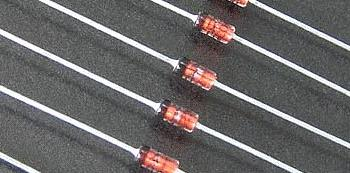A Zener Diode is a special kind of diode which permits current to flow in the forward direction as normal, but will also allow it to flow in the reverse direction when the voltage is above a certain value – the breakdown voltage known as the Zener voltage.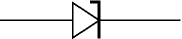The Zener voltage of a standard diode is high, but if a reverse current above that value is allowed to pass through it, the diode is permanently damaged. Zener diodes are designed so that their zener voltage is much lower – for example just 2.4 Volts. When a reverse current above the Zener voltage passes through a Zener diode, there is a controlled breakdown which does not damage the diode. The voltage drop across the Zener diode is equal to the Zener voltage of that diode no matter how high the reverse bias voltage is above the Zener voltage.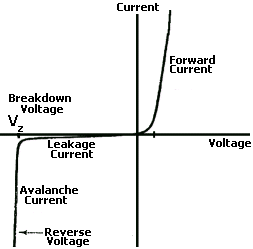The illustration above shows this phenomenon in a Current vs. Voltage graph. With a zener diode connected in the forward direction, it behaves exactly the same as a standard diode – i.e. a small voltage drop of 0.3 to 0.7V with current flowing through pretty much unrestricted. In the reverse direction however there is a very small leakage current between 0V and the Zener voltage – i.e. just a tiny amount of current is able to flow. Then, when the voltage reaches the breakdown voltage (Vz), suddenly current can flow freely through it. For example if you pass a reverse 5V through a 3V zener diode and measure the voltage across the zener diode, that voltage will be 3V.

### Uses of Zener Diodes

Since the voltage dropped across a Zener Diode is a known and fixed value, Zener diodes are typically used to regulate the voltage in electric circuits. Using a resistor to ensure that the current passing through the Zener diode is at least 5mA (0.005 Amps), the circuit designer knows that the voltage drop across the diode is exactly equal to the Zener voltage of the diode.

NEW Click here to visit Zener-Diode.co.uk for a site dedicated to zener diodes, their characteristics, usage, and other information.

### Zener Diode Voltage Regulator Circuit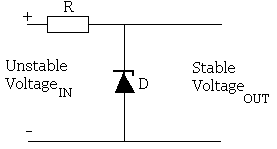A zener diode can be used to make a simple voltage regulation circuit as pictured above. The output voltage is fixed as the zener voltage of the zener diode used and so can be used to power devices requiring a fixed voltage of a certain value (equal to the rating of the zener diode). Click here to find out more about the Zener Diode Voltage Regulator and how you go about selecting the resistor which burns off the excess voltage as heat, and zener diode.
NEW The above mentioned article on zener diode voltage regulators has now been updated and a handy calculator has been added to help you select the correct resistor (resistance value and power rating) and zener diode value and power rating.

### Zener Diodes in Series With Loads

If you have a regulated fixed voltage – say 12 Volts from a desktop PC power supply, and you want to power something requiring a lower voltage, it is possible to simply place a zener diode in series with the load device. You would choose a diode with a zener voltage equal to the supply voltage minus the voltage drop across the load.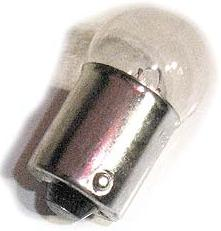For example, if you have a 1 Watt 6 Volt lightbulb to power from a 12 Watt regulated power supply, a 6.2V zener diode could be placed in reverse in series with the bulb leaving the bulb with 5.8V with the remaining 6.2V lost as heat through the zener diode (which would need to be rated at over 1 Watt). Alternatively you could overpower the bulb a little using a 5.6V zener diode giving 6.4V to the bulb. Heatwould be generated in the zener diode so it is essential to calculate the power loss in it so a suitably rated diode could be chosen.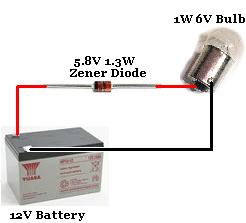This is illustrated in the circuit diagram above. Note the orientation of the zener diode.

Using a 5.6V zener diode, and knowing the bulb draws a current of around one-sixth of an Amp, we can calculate the power loss in the diode (with Ohm’s Law) to be just under 1 Watt. Therefore a standard1.3 Watt zener diode will be up to the job.

### Circuits with Zener Diodes

Zener diodes are key components in the circuits presented in the following REUK.co.uk articles: Make a Simple Battery Status Monitor, LM741 Voltage Indicator, and High Capacity Alternative to 9V Battery.